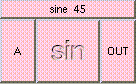SINETHE BOX'S MAIN HELP: This function returns a float between [-1,1], the sine of angle A. A (input): This must be a float. This is the angle in degrees. OUT (output): This function returns a float between [-1,1], the sine of angle A.

<-- BACK TO Math